Meta  0.1 A tiny metaprogramming libraryTransformation

## Description

Transformation algorithms.

lazy

## Typedefs

template<typename List , typename State , typename Fun >
using meta::accumulate = fold< List, State, Fun >
An alias for meta::fold. More...

template<typename ListOfLists >
using meta::cartesian_product = reverse_fold< ListOfLists, list< list<>>, quote_trait< detail::cartesian_product_fn >>
Given a list of lists ListOfLists, return a new list of lists that is the Cartesian Product. Like the sequence function from the Haskell Prelude. More...

template<typename... Lists>
using meta::concat = _t< detail::concat_< Lists... >>
Concatenates several lists into a single list. More...

template<typename List , typename N >
using meta::drop = _t< detail::drop_< List, N >>
Return a new meta::list by removing the first N elements from List. More...

template<typename List , std::size_t N>
using meta::drop_c = _t< detail::drop_< List, meta::size_t< N >>>
Return a new meta::list by removing the first N elements from List. More...

template<typename List , typename Pred >
using meta::filter = fold< List, list<>, detail::filter_< Pred >>
Returns a new meta::list where only those elements of List that satisfy the Callable Pred such that invoke<Pred,A>::value is true are present. That is, those elements that don't satisfy the Pred are "removed". More...

template<typename List , typename State , typename Fun >
using meta::fold = _t< detail::fold_< List, State, Fun >>
Return a new meta::list constructed by doing a left fold of the list List using binary Callable Fun and initial state State. That is, the State_N for the list element A_N is computed by Fun(State_N-1, A_N) -> State_N. More...

template<typename ListOfLists >
using meta::join = apply< quote< concat >, ListOfLists >
Joins a list of lists into a single list. More...

template<typename List , typename Pred >
using meta::partition = fold< List, pair< list<>, list<>>, detail::partition_< Pred >>
Returns a pair of lists, where the elements of List that satisfy the Callable Pred such that invoke<Pred,A>::value is true are present in the first list and the rest are in the second. More...

template<typename List >
using meta::pop_front = _t< detail::pop_front_< List >>
Return a new meta::list by removing the first element from the front of List. More...

template<typename List , typename T >
using meta::push_back = _t< detail::push_back_< List, T >>
Return a new meta::list by adding the element T to the back of List. More...

template<typename List , typename T >
using meta::push_front = _t< detail::push_front_< List, T >>
Return a new meta::list by adding the element T to the front of List. More...

template<typename List , typename T , typename U >
using meta::replace = _t< detail::replace_< List, T, U >>
Return a new meta::list where all instances of type T have been replaced with U. More...

template<typename List , typename C , typename U >
using meta::replace_if = _t< detail::replace_if_< List, C, U >>
Return a new meta::list where all elements A of the list List for which invoke<C,A>::value is true have been replaced with U. More...

template<typename List >
using meta::reverse = reverse_fold< List, list<>, quote< push_back >>
Return a new meta::list by reversing the elements in the list List. More...

template<typename List , typename State , typename Fun >
using meta::reverse_fold = _t< detail::reverse_fold_< List, State, Fun >>
Return a new meta::list constructed by doing a right fold of the list List using binary Callable Fun and initial state State. That is, the State_N for the list element A_N is computed by Fun(A_N, State_N+1) -> State_N. More...

template<typename List , typename Pred >
using meta::sort = _t< detail::sort_< List, Pred >>
Return a new meta::list that is sorted according to Callable predicate Pred. More...

template<typename... Args>
using meta::transform = _t< detail::transform_< list< Args... >>>
Return a new meta::list constructed by transforming all the elements in List with the unary Callable Fun. transform can also be called with two lists of the same length and a binary Callable, in which case it returns a new list constructed with the results of calling Fun with each element in the lists, pairwise. More...

template<typename ListOfLists >
using meta::transpose = fold< ListOfLists, repeat_n< size< front< ListOfLists >>, list<>>, bind_back< quote< transform >, quote< push_back >>>
Given a list of lists of types ListOfLists, transpose the elements from the lists. More...

template<typename List >
using meta::unique = fold< List, list<>, quote_trait< detail::insert_back_ >>
Return a new meta::list where all duplicate elements have been removed. More...

template<typename ListOfLists >
using meta::zip = transpose< ListOfLists >
Given a list of lists of types ListOfLists, construct a new list by grouping the elements from the lists pairwise into meta::lists. More...

template<typename Fun , typename ListOfLists >
using meta::zip_with = transform< transpose< ListOfLists >, uncurry< Fun >>
Given a list of lists of types ListOfLists and a Callable Fun, construct a new list by calling Fun with the elements from the lists pairwise. More...

## Typedef Documentation

template<typename List , typename State , typename Fun >
 using meta::accumulate = typedef fold

#include <meta/meta.hpp>

An alias for meta::fold.

Complexity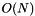.

Definition at line 1050 of file meta.hpp.

template<typename ListOfLists >
 using meta::cartesian_product = typedef reverse_fold>, quote_trait>

#include <meta/meta.hpp>

Given a list of lists ListOfLists, return a new list of lists that is the Cartesian Product. Like the sequence function from the Haskell Prelude.

Complexity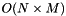, where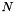is the size of the outer list, and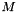is the size of the inner lists.

Definition at line 2869 of file meta.hpp.

template<typename... Lists>
 using meta::concat = typedef _t>

#include <meta/meta.hpp>

Concatenates several lists into a single list.

Precondition
The parameters must all be instantiations of meta::list.
Complexity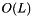where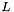is the number of lists in the list of lists.

Definition at line 1190 of file meta.hpp.

template<typename List , typename N >
 using meta::drop = typedef _t>

#include <meta/meta.hpp>

Return a new meta::list by removing the first N elements from List.

Complexity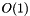.

Definition at line 1367 of file meta.hpp.

template<typename List , std::size_t N>
 using meta::drop_c = typedef _t>>

#include <meta/meta.hpp>

Return a new meta::list by removing the first N elements from List.

Complexity.

Definition at line 1374 of file meta.hpp.

template<typename List , typename Pred >
 using meta::filter = typedef fold, detail::filter_>

#include <meta/meta.hpp>

Returns a new meta::list where only those elements of List that satisfy the Callable Pred such that invoke<Pred,A>::value is true are present. That is, those elements that don't satisfy the Pred are "removed".

Complexity.

Definition at line 2058 of file meta.hpp.

template<typename List , typename State , typename Fun >
 using meta::fold = typedef _t>

#include <meta/meta.hpp>

Return a new meta::list constructed by doing a left fold of the list List using binary Callable Fun and initial state State. That is, the State_N for the list element A_N is computed by Fun(State_N-1, A_N) -> State_N.

Complexity.

Definition at line 1043 of file meta.hpp.

template<typename ListOfLists >
 using meta::join = typedef apply, ListOfLists>

#include <meta/meta.hpp>

Joins a list of lists into a single list.

Precondition
The parameter must be an instantiation of meta::list<T...> where each T is itself an instantiation of meta::list.
Complexitywhereis the number of lists in the list of lists.

Definition at line 1208 of file meta.hpp.

template<typename List , typename Pred >
 using meta::partition = typedef fold, list<>>, detail::partition_>

#include <meta/meta.hpp>

Returns a pair of lists, where the elements of List that satisfy the Callable Pred such that invoke<Pred,A>::value is true are present in the first list and the rest are in the second.

Complexity.

Definition at line 2417 of file meta.hpp.

template<typename List >
 using meta::pop_front = typedef _t>

#include <meta/meta.hpp>

Return a new meta::list by removing the first element from the front of List.

Complexity.

Definition at line 1506 of file meta.hpp.

template<typename List , typename T >
 using meta::push_back = typedef _t>

#include <meta/meta.hpp>

Return a new meta::list by adding the element T to the back of List.

Complexity.
Note
pop_back not provided because it cannot be made to meet the complexity guarantees one would expect.

Definition at line 1541 of file meta.hpp.

template<typename List , typename T >
 using meta::push_front = typedef _t>

#include <meta/meta.hpp>

Return a new meta::list by adding the element T to the front of List.

Complexity.

Definition at line 1473 of file meta.hpp.

template<typename List , typename T , typename U >
 using meta::replace = typedef _t>

#include <meta/meta.hpp>

Return a new meta::list where all instances of type T have been replaced with U.

Complexity.

Definition at line 1902 of file meta.hpp.

template<typename List , typename C , typename U >
 using meta::replace_if = typedef _t>

#include <meta/meta.hpp>

Return a new meta::list where all elements A of the list List for which invoke<C,A>::value is true have been replaced with U.

Complexity.

Definition at line 1936 of file meta.hpp.

template<typename List >
 using meta::reverse = typedef reverse_fold, quote>

#include <meta/meta.hpp>

Return a new meta::list by reversing the elements in the list List.

Complexity.

Definition at line 2211 of file meta.hpp.

template<typename List , typename State , typename Fun >
 using meta::reverse_fold = typedef _t>

#include <meta/meta.hpp>

Return a new meta::list constructed by doing a right fold of the list List using binary Callable Fun and initial state State. That is, the State_N for the list element A_N is computed by Fun(A_N, State_N+1) -> State_N.

Complexity.

Definition at line 1097 of file meta.hpp.

template<typename List , typename Pred >
 using meta::sort = typedef _t>

#include <meta/meta.hpp>

Return a new meta::list that is sorted according to Callable predicate Pred.

Complexity
Expected: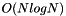Worst case: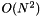.
using L0 = list<char, char, char, char, char, char, char>;
static_assert(std::is_same<L1, list<char, char, char, char, char, char, char>>::value, "");

Definition at line 2474 of file meta.hpp.

template<typename... Args>
 using meta::transform = typedef _t>>

#include <meta/meta.hpp>

Return a new meta::list constructed by transforming all the elements in List with the unary Callable Fun. transform can also be called with two lists of the same length and a binary Callable, in which case it returns a new list constructed with the results of calling Fun with each element in the lists, pairwise.

Complexity.

Definition at line 2027 of file meta.hpp.

template<typename ListOfLists >
 using meta::transpose = typedef fold>, list<>>, bind_back, quote>>

#include <meta/meta.hpp>

Given a list of lists of types ListOfLists, transpose the elements from the lists.

Complexity, whereis the size of the outer list, andis the size of the inner lists.

Definition at line 2125 of file meta.hpp.

template<typename List >
 using meta::unique = typedef fold, quote_trait>

#include <meta/meta.hpp>

Return a new meta::list where all duplicate elements have been removed.

Complexity.

Definition at line 2375 of file meta.hpp.

template<typename ListOfLists >
 using meta::zip = typedef transpose

#include <meta/meta.hpp>

Given a list of lists of types ListOfLists, construct a new list by grouping the elements from the lists pairwise into meta::lists.

Complexity, whereis the size of the outer list, andis the size of the inner lists.

Definition at line 2163 of file meta.hpp.

template<typename Fun , typename ListOfLists >
 using meta::zip_with = typedef transform, uncurry>

#include <meta/meta.hpp>

Given a list of lists of types ListOfLists and a Callable Fun, construct a new list by calling Fun with the elements from the lists pairwise.

Complexity, whereis the size of the outer list, andis the size of the inner lists.

Definition at line 2144 of file meta.hpp.